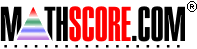Math Practice Online > free > lessons > Florida > 6th grade > Proportions 2

## Proportions 2

This topic covers proportions of shapes.

 Sample Problems for Proportions 2 Lesson for Proportions 2

### This topic aligns to the following state standards

Grade 8: Num 1. Knows the appropriate operations to solve real-world problems involving integers, ratios, rates, proportions, numbers expressed as percents, decimals, and fractions.
Grade 8: Num 2. Solves real-world problems involving integers, ratios, proportions, numbers expressed as percents, decimals, and fractions in two- or three-step problems.
Grade 6: Meas 1. Knows proportional relationships in scale drawings.
Grade 7: Meas 2. Knows proportional relationships used in scale drawings.
Grade 8: Meas 1. Interprets and applies various scales including those based on number lines, graphs, models, and maps. (Scale may include rational numbers.)
Grade 7: Meas 3. Finds measures of length, weight or mass, and capacity or volume using proportional relationships and properties of similar geometric figures (for example, using shadow measurement and properties of similar triangles to find the height of a flag pole).
Grade 8: Meas 1. Finds measures of length, weight or mass, and capacity or volume using proportional relationships and properties of similar geometric figures.
Grade 9: Geo 1. represents and applies geometric properties and relationships to solve real-world and mathematical problems including ratio, proportion, and properties of right triangle trigonometry

Copyright Accurate Learning Systems Corporation 2008.